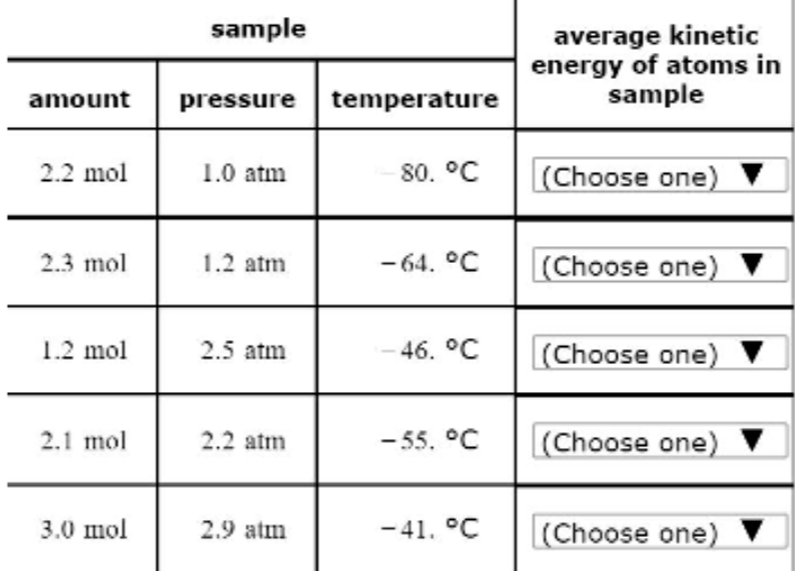# Problem: Five samples of helium gas are described in the table below. Rank the samples in order of increasing average kinetic energy of the atoms in them. That is, select "1" next to the sample in which the helium atoms have the lowest average kinetic energy. Select "2" next to the sample in which the helium atoms have the next lowest average kinetic energy, and so on.

97% (71 ratings)
###### FREE Expert Solution
97% (71 ratings)###### Problem Details

Five samples of helium gas are described in the table below. Rank the samples in order of increasing average kinetic energy of the atoms in them. That is, select "1" next to the sample in which the helium atoms have the lowest average kinetic energy. Select "2" next to the sample in which the helium atoms have the next lowest average kinetic energy, and so on.What scientific concept do you need to know in order to solve this problem?

Our tutors have indicated that to solve this problem you will need to apply the Kinetic Molecular Theory concept. You can view video lessons to learn Kinetic Molecular Theory. Or if you need more Kinetic Molecular Theory practice, you can also practice Kinetic Molecular Theory practice problems.

What is the difficulty of this problem?

Our tutors rated the difficulty ofFive samples of helium gas are described in the table below....as medium difficulty.

How long does this problem take to solve?

Our expert Chemistry tutor, Dasha took 1 minute and 56 seconds to solve this problem. You can follow their steps in the video explanation above.

What professor is this problem relevant for?

Based on our data, we think this problem is relevant for Professor Toole's class at UTOLEDO.